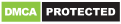# Python 3.7 Matplotlib — Data Visualization Tutorial

Matplotlib is a python library that allows you to represent your data visually. It's particularly useful for data science and machine learning developers.

Matplotlib is the most visualization package for Python. You can use to draw charts in your Python scripts, the Python interactive shells, the Jupyter notebook, or your backend web applications built on Python (e.g. Django or Flask etc. ).

In this tutorial, we'll see by example how to create a simple Python 3.7 application which uses `matpotlib` to plot your data charts.

## Prerequisites

We'll be creating a Python 3.7 application so you need to have a few prerequisites:

• Your development environment should have Python 3.7 installed with `pip` and `venv`.
• You need to have a working knowledge of Python.

## Creating a Virtual Environment

For isolating your current project packages from the other Python packages installed on your system, you need to create an isolated virtual environment using `venv`:

``````\$ python -m venv env
``````

Next, activate your environment using the `source` command:

``````\$ source env/bin/activate
``````

On Windows, you need to use the following script instead:

``````\$ source env/Scripts/activate
``````

## Installing `matplotlib`

You can now install `matplotlib` in Python 3 from PyPI using `pip` by running the following command:

``````\$ pip install matplotlib
``````

As of this writing, matplotlib v3.0.2 will be installed. Also many other libraries will be automatically installed as dependencies of `matplotlib`.

You can run `pip freeze` to see all the installed dependencies with their versions:

``````cycler==0.10.0
kiwisolver==1.0.1
matplotlib==3.0.2
numpy==1.15.4
pyparsing==2.3.0
python-dateutil==2.7.5
six==1.12.0
``````

One of the dependencies of `matplotlib` is `numpy`.

NumPy is a popular Python library for scientific computing which is available under the BSD license. It provides many features such as:

• Working with powerful N-dimensional arrays,
• Easy integration with C/C++ and Fortran,
• Versatile APIs for advanced math like linear algebra, Fourier transform, and random numbers etc.

Numpy is not just for scientific applications. It can be used as a container for multi-dimensional data which makes it integrate well with many databases.

## How to Use `matplotlib`?

Generally, you can follow these steps to create visualizations with `matplotlib`:

• First of all, you need to choose the right chart type for representing your data,
• Next, you need to provide a title and labels for the plot axis,
• Next, customize different aspects like size and colors,
• Finally plot your data.

## Drawing Charts with the `pyplot` Package

Let's suppose, you want to draw the `f(x) = x*x` mathematical function. We simpy need to import the necessary `matplotlib` API and follow simple instruction to draw the chart.

In this example, we'll be using the`pyplot` object.

`pyplot` provides a set of commands that makes `matplotlib` work like MATLAB.

Let's see a simple example from the Python IDE:

``````\$ python
Python 3.7.0 (v3.7.0:1bf9cc5093, Jun 27 2018, 04:06:47) [MSC v.1914 32 bit (Intel)] on win32
``````

First import `matplotlib.pyplot`

``````>>> import matplotlib.pyplot as pyplot
``````

Next import `numpy`:

``````>>> import numpy as np
``````

We'll be using `numpy` for declaring a data container that holds the data we want to draw.

Next, you need to create a figure and an axes:

``````>>> figure = pyplot.figure()
>>> axes = pyplot.axes()
``````

Next, create a sequence of numbers using the `linespace()` method of `numpy`.

``````x = np.linspace(0, 10, 1000)
axes.plot(x, x*x);
``````

Next, you can show the chart using the `show()` command:

``````>>> pyplot.show()
``````

A window will be opened with your chart:# About the Author

Techiediaries is a website dedicated to bring you tutorials for the latest web technologies

## Read our other tutorials